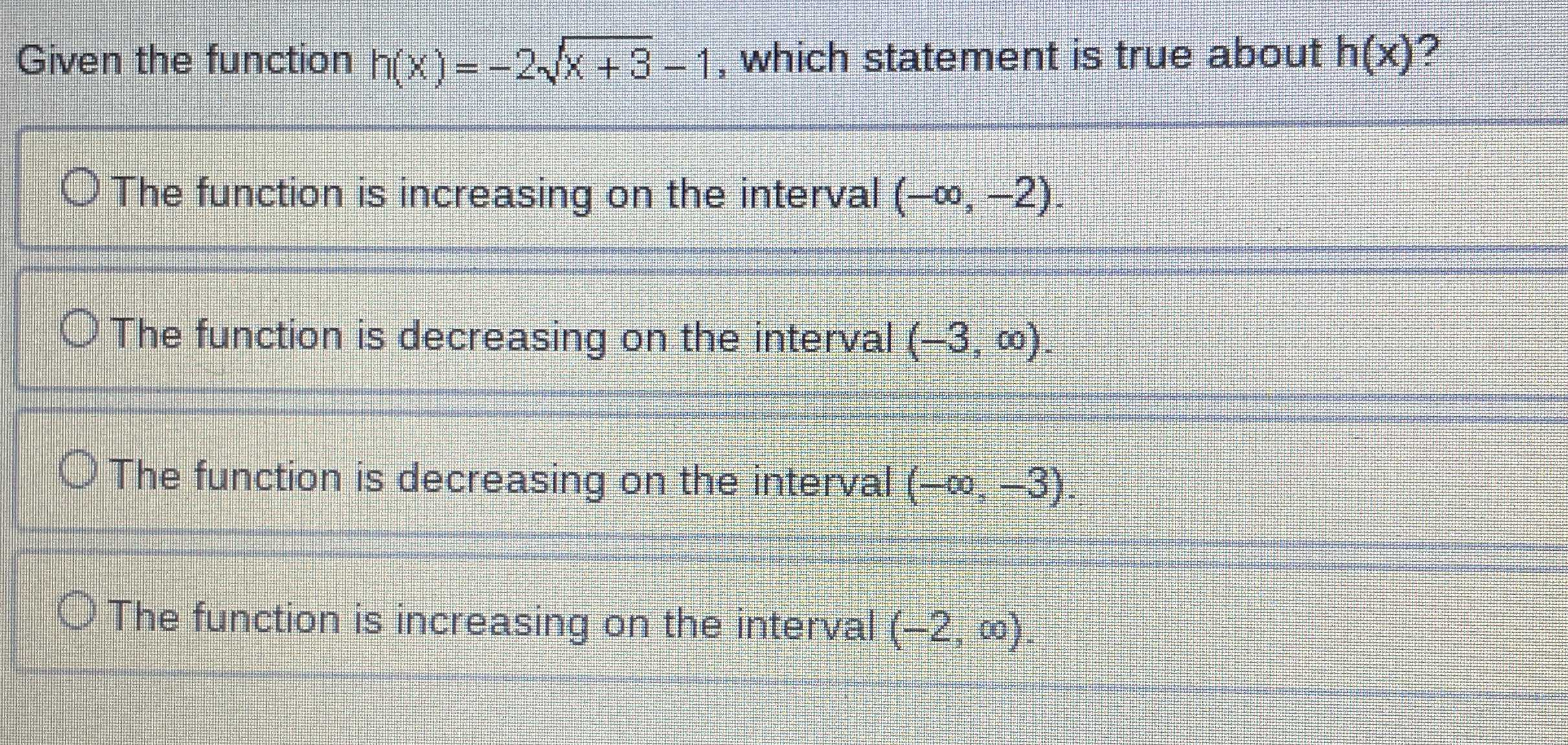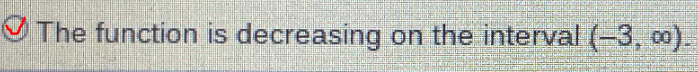### Still have math questions?

Algebra
QuestionGiven the function $$h ( x ) = - 2 \sqrt { x + 3 } - 1$$ , which statement is true about $$h ( x )$$ ?

The function is increasing on the interval $$( - \infty , - 2 )$$ .

The function is decreasing on the interval $$( - 3 , \infty )$$

The function is decreasing on the interval $$( - \infty , - 3 )$$ .

The function is increasing on the interval $$( - 2 , \infty )$$ .